# AP Chemistry : Gibbs Free Energy and Spontaneity

## Example Questions

← Previous 1

### Example Question #1 : Gibbs Free Energy And Spontaneity

Suppose that a rxn has ∆H = -28 kJ and ∆S= -60 J/K. At what temperature will it
change from spontaneous to non-spontaneous?

46.7 K

50

467 K

500 K

467 K

Explanation:

Approximately 467 K. ∆G=∆H-T∆S and a rxn proceeds spontaneously when ∆G < 0
and is non-spontaneous when ∆G > 0. So if we set ∆G=0 and solve the equation for T, we will see that the crossover from spontaneous to non-spontaneous occurs when T=467K.

### Example Question #1 : Gibbs Free Energy And Spontaneity

If the reaction quotient (Q) is greater than the equilibrium constant (K), what is true about the Gibbs free energy?

It is greater than zero.

It is equal to zero.

It is less than zero.

It is greater than zero.

Explanation:

If Q is greater than K, the reaction has exceeded the equilibrium state. It will proceed nonspontaneously (since equilibrium has already been reached), and this must mean that the ΔG (gibbs free energy) must be positive, or greater than zero.

### Example Question #1 : Gibbs Free Energy And Spontaneity

The entropy and enthalpy of a reaction are both negative. Is the reaction spontaneous?

The reaction will be spontaneous if and only if the magnitude of the enthalpy is greater than the magnitude of the entropy times the temperature

The reaction is not spontaneous

The reaction will be spontaneous if and only if the magnitude of the entropy is greater than the magnitude of the enthalpy times the temperature

The reaction is spontaneous

The reaction will be spontaneous if and only if the magnitude of the enthalpy is greater than the magnitude of the entropy

The reaction will be spontaneous if and only if the magnitude of the enthalpy is greater than the magnitude of the entropy times the temperature

Explanation:

A reaction is spontaneous if the Gibb's Free Energy of the reaction is negative.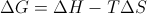If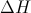, the enthalpy, and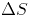, the entropy, are both negative, then the reaction will be spontaneous if and only if the magnitude of the enthalpy is greater than the magnitude of the entropy times the temperature.

### Example Question #1 : Gibbs Free Energy And Spontaneity

A system with __________ and __________ will never be spontaneous.

negative enthalpy . . . positive entropy

positive enthalpy . . . negative entropy

positive enthalpy . . . positive entropy

negative enthalpy . . . negative entropy

positive enthalpy . . . negative entropy

Explanation:

The Gibb's free energy equation is used to determine the spontaneity of a reaction and is written as follows:.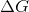is Gibb's free energy,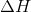is enthalpy, and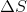is entropy. In order for a reaction to be spontaneous, Gibb's free energy must have a negative value. Based on the equation, we can see that a positive enthalpy in combination with a negative entropy will always result in a positive value for Gibb's free energy.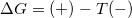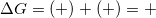This means these are the conditions that will always result in a nonspontaneous reaction.

### Example Question #5 : Gibbs Free Energy And Spontaneity

A chemical reaction has the following changes in enthalpy and entropy.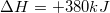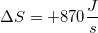What is the temperature range for this reaction that allow it to be spontaneous?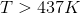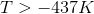The reaction is always spontaneous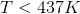Explanation:

A reaction is spontaneous when Gibb's free energy is negative. As a result, we need to determine the temperature range where Gibb's free energy is less than zero. Since we know the values for changes in enthalpy and entropy, we can plug them into the Gibb's free energy equation, and set it equal to zero.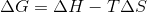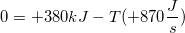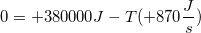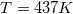437K is the temperature at which Gibb's free energy is zero. Since entropy is positive for this reaction, increasing the temperature will result in a more negative value for Gibb's free energy.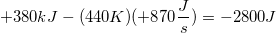As a result, any temperature that is greater than 437K will make this reaction spontaneous.

### Example Question #1 : Gibbs Free Energy And Spontaneity

Consider the following reaction in a galvanic cell: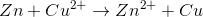Which if the following statements about the reaction is false?

The reaction is spontaneous

The equilibrium constant is greater than one

The cell potential is positive

Gibb's free energy is positive

Gibb's free energy is positive

Explanation:

A galvanic cell results in a positive cell potential from a spontaneous reaction. Spontaneous reactions always have a negative Gibb's free energy.

An equilibrium constant greater than one would indicate that the equilibrium concentration of products is greater than the equilibrium concentration of reactants, consistent with a spontaneous reaction.

### Example Question #1 : Gibbs Free Energy And Spontaneity

Consider the following reaction in a galvanic cell: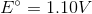What is the Gibb's free energy for the reaction under standard conditions?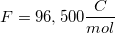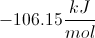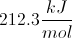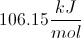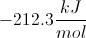Explanation:

You can find the Gibb's free energy of a galvanic cell by using the following equation: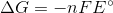is the number of moles of electrons that are transferred in the reaction,is Faraday's constant, and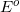is the potential of the cell.

We are given the constant value and the cell potential. The moles of electrons transferred is equal to the change in charge on the atoms in the reaction. In this reaction, copper is reduced from a charge of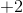to zero, requiring that is gained two moles of electrons.

Using these values, we can find Gibb's free energy for the cell.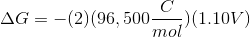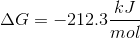Notice that the value is negative. Galvanic cells are spontaneous, and will have negative Gibb's free energies at standard conditions.

### Example Question #8 : Gibbs Free Energy And Spontaneity

If the reaction quotient (Q) is less than the equilibrium constant (K), what is true about the Gibbs free energy?

We must know the reaction enthalpy to determine the answer

It is greater than zero

It is equal to zero

We must know the reaction temperature to determine the answer

It is less than zero

It is less than zero

Explanation:

If Q is less than K, then the reaction has not yet reached the equilibrium state. It will proceed spontaneously in the forward direction. Since it is proceeding spontaneously in the forward direction, this must mean that the ΔG (Gibbs free energy) must be negative, or less than zero.

Enthalpy and temperature are necessary when mathematically evaluating Gibbs free energy, but the reaction quotient and equilibrium constant provide enough information to qualitatively determine that the reaction is proceeding spontaneously.

### Example Question #9 : Gibbs Free Energy And Spontaneity

Of the following reactions, which of the following is only spontaneous at high enough temperatures?

∆H –, ∆S +

∆H –, ∆S –

∆H +, ∆S –

None of the Above

∆H +, ∆S +

∆H +, ∆S +

Explanation:

Based on Gibbs Free Energy, ∆G = ∆H – T∆S, we find that ∆H– will contribute to spontaneity (∆G<0). Since we are looking at temperatures, in order for ∆S to make an nonspontaneous reaction spontaneous at only high temperatures, ∆H will be positive, and ∆S will be positive.

### Example Question #1 : Gibbs Free Energy And Spontaneity

If a reaction has a positive value for its enthalpy and a negative value for its entropy, which of the following is true?

Not enough information to tell
The reaction is spontaneous
The reaction is nonspontaneous
The reaction is at equilibrium
Correct answer: The reaction is nonspontaneous
Explanation:

Given the equation for free energy, (delta)G = (delta)H-T(delta)S, we can determine that the reaction is nonspontaneous at all temperatures if H is positive and S is negative. This combination would always lead to a positive G value, meaning that free energy is required for the reaction to take place and it is therefore nonspontaneous.

← Previous 1

### All AP Chemistry Resources